# Colleges with the highest SAT scores in California

Top 10 colleges in California with the highest SAT scores
Looking for the colleges with the highest SAT scores in California? Well you're in luck! We've compiled a national college database and have created a list of the top 10 universities with the highest SAT scores in California. These are the schools whose applicants had the highest average SAT scores in California. And, since these tests are meant to determine academic prowess, they are arguably the schools with the most academically proficient students. You could even say these are the best colleges in California. We also include each college's ACT scores and acceptance rate so that you can see where you would have the easiest or hardest time getting in. Read on to find out more.

## California Institute of Technology SAT scores

The average SAT score for California Institute of Technology is 1545.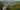The average SAT score of 1545 breaks down into:

• SAT math: 795

The average ACT score for California Institute of Technology is 36 and their acceptance rate is 6.4%.

## Harvey Mudd College SAT scores

The average SAT score for Harvey Mudd College is 1530.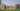The average SAT score of 1530 breaks down into:

• SAT math: 790

The average ACT score for Harvey Mudd College is 35 and their acceptance rate is 14.5%.

## Stanford University SAT scores

The average SAT score for Stanford University is 1505.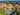The average SAT score of 1505 breaks down into:

• SAT math: 770

The average ACT score for Stanford University is 34 and their acceptance rate is 4.3%.

## Pomona College SAT scores

The average SAT score for Pomona College is 1465.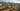The average SAT score of 1465 breaks down into:

• SAT math: 745

The average ACT score for Pomona College is 34 and their acceptance rate is 7.4%.

## University of Southern California SAT scores

The average SAT score for University of Southern California is 1440.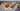The average SAT score of 1440 breaks down into:

• SAT math: 740

The average ACT score for University of Southern California is 32 and their acceptance rate is 13%.

## Claremont McKenna College SAT scores

The average SAT score for Claremont McKenna College is 1427.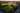The average SAT score of 1427 breaks down into:

• SAT math: 730

The average ACT score for Claremont McKenna College is 32 and their acceptance rate is 9.3%.

## Berkeley University of California SAT scores

The average SAT score for Berkeley University of California is 1415.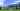The average SAT score of 1415 breaks down into:

• SAT math: 725

The average ACT score for Berkeley University of California is 31 and their acceptance rate is 14.8%.

## Pitzer College SAT scores

The average SAT score for Pitzer College is 1410.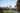The average SAT score of 1410 breaks down into:

• SAT math: 720

The average ACT score for Pitzer College is 31 and their acceptance rate is 13.3%.

## University of California Los Angeles SAT scores

The average SAT score for University of California Los Angeles is 1405.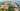The average SAT score of 1405 breaks down into:

• SAT math: 715

The average ACT score for University of California Los Angeles is 31 and their acceptance rate is 12.3%.

## Scripps College SAT scores

The average SAT score for Scripps College is 1390.The average SAT score of 1390 breaks down into:

• SAT math: 690